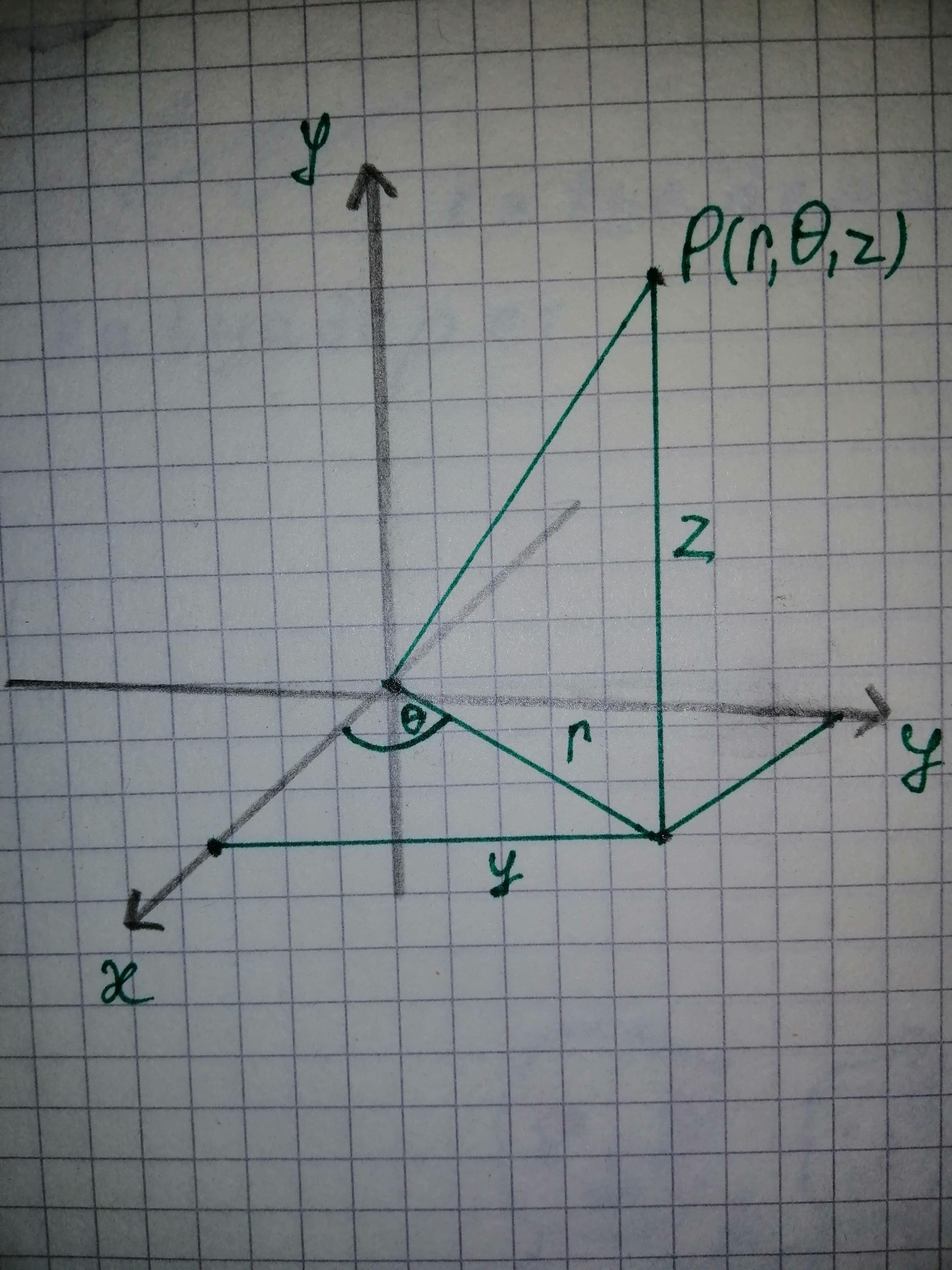# How are triple integrals defined in cylindrical and spherical coordinates? Why might one prefer working in one of these coordinate systems to working in rectangular coordinates?ossidianaZ 2021-01-06 Answered
How are triple integrals defined in cylindrical and spherical coordinates?
Why might one prefer working in one of these coordinate systems to working in rectangular coordinates?
You can still ask an expert for help

• Questions are typically answered in as fast as 30 minutes

Solve your problem for the price of one coffee

• Math expert for every subject
• Pay only if we can solve itBertha Stark

Step 1
The equation relating to rectangular (x, y, z) and cylindrical $\left(r,\theta ,z\right)$ coordinates are, $x=r\mathrm{cos}\theta ,y=\mathrm{sin}\theta ,z=z$
${r}^{2}={x}^{2}+{y}^{2}$
Here, $\mathrm{tan}\theta =\frac{y}{x}$
Hence , the solution is $\mathrm{tan}\theta =\frac{y}{x}.$
The equation relating spherical coordinates to Cartesian and cylindrical coordinates is, $\text{Undefined control sequence \cancel}$
$\text{Undefined control sequence \cancel}$
Step 2
Here, $\delta =\sqrt{{x}^{2}+{y}^{2}+{z}^{2}}$
From equation 1, we know ${x}^{2}+{y}^{2}={r}^{2}.$
Therefore,
$\delta =\sqrt{{r}^{2}+{z}^{2}}$
Hence, the solution is $\delta =\sqrt{{r}^{2}+{x}^{2}}.$
The diagram regarding the cylindrical coordinates $\left(r,\theta ,z\right)$ is given below: